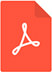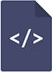# The simplest correction to Hubble’s law and its theoretical implicationsPDF Full Text

# Abstract

This paper introduces a simple change to the Hubble equation on distance to red shift. It is shown that the prediction of the equation is compatible with data, obtained from distance measurements, which are independent of red shift. Having shown its compatibility with these observations, the paper then introduces the theoretical basis for the equation. The theoretical basis relies on a single assumption that in the expansion of the universe, the ratio d(space)/d(time) = Constant = speed of light. It is shown that the equations of special relativity follow directly from this 1 assumption.

# Keywords

Hubble’s law, theoretical implications